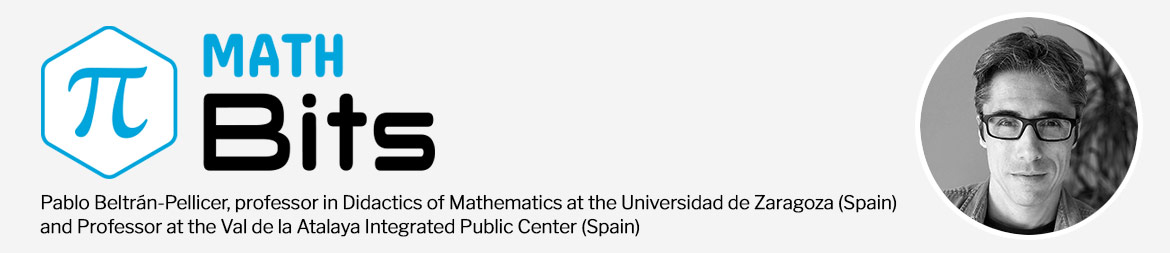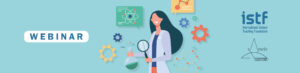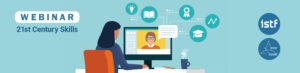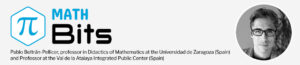# Teaching and Learning Integers in Math BitsIf we reflect on the common word problems on integers that students do in grade 7, it is a little worrisome to realize that the average third or fourth grader in Primary School could solve them too. These problems, or rather exercises, involve real-world contexts such as debts and assets, elevators, depths to which a diver descends, temperatures, ages of historical figures, points won or lost in a game, and so on.

Do we need to use the complete notation (with signed numbers in parentheses) to solve these problems? Is it necessary to have a knowledge of integers as a numerical structure to be able to address them? Moreover, are we maybe implying that ancient Romans, who certainly did not use integers, didn’t know how to solve problems involving debts and assets?

Integers pose a real challenge to teachers. They are still deeply rooted today for no apparent reason, as a sort of remnant left of modern mathematics. In the 1960s and 70s this trend aimed to base school mathematics on the theory of sets and algebraic structures. Terms such as applications, equivalence classes, and properties were customary in textbooks, and in some countries they were used through much of the early 1980s. So integers were presented as a set of already constructed numbers that had certain properties.

The obstacles that students encounter when dealing with integers can be traced back to the origins of these numbers. This informs us that the difficulties are not merely cognitive but related to the nature of the mathematical object in question. Although certain forms of “negativity” are already found in ancient Chinese mathematics or in Diophantus’ Arithmetica, the study of negative numbers is a history fraught with obstacles. Some examples include:

• Difficulty in manipulating isolated negative amounts. (Diophantus formulates the rule of signs, but he doesn’t recognize isolated negative amounts.)
• Difficulty in giving meaning to isolated negative amounts (Stevin, D’Alembert, Carnot, perhaps Descartes).
• Difficulty in unifying the real number line (since positive and negative numbers were conceived as objects of different categories that were neutralized; Maclaurin, D’Alembert, Carnot, and Cauchy).
• Dual nature of zero. (How can something be less than nothing? Stevin, Maclaurin, Carnot, Cauchy, and perhaps also Euler and Laplace).
• Stagnation at the stage of concrete operations (the additive structure is assumed, but not the multiplicative one).

The proposal by Math Bits is an adaptation of the proposal by Eva Cid, a researcher in mathematics didactics specialized in integers. She picks up the gauntlet thrown down by the research community a long time ago, which has long acknowledged that there exists no concrete model that allows its properties to be thoroughly abstracted. The commonly used models, such as debts and assets, only allow us to justify the additive structure, albeit not entirely satisfactorily.

When we as teachers think that teaching negative whole numbers using debts and assets is useful is because we already know what their structure is like, and we look for arguments that conform to it. The following example shows the reasoning used by a student, which is totally legitimate:

• Student: (+70) − (−10) = + 70 because if I have € 70 and my € 10 debt is forgiven, I still have € 70.
• Teacher: No. Having € 70 and not carrying debt is equivalent to having € 80 and owing € 10. If now the € 10 debt is forgiven, we have an asset of € 80, then (+70) − (−10) = + 80.

Similar things happen with just any specific model that comes to mind. We can group them into two categories:

• Neutralization models. They are based on the manipulation of quantities having opposite directions that cancel out. To describe them, we will use a two-color counter model where the colored counters of two different colors cancel each other out. Examples: debts and assets; people entering or leaving an area; games with positive or negative scores; two-color counters that cancel each other out; positive or negative electrical charges, etc.
• Displacement models. Given an origin, positive or negative numbers can indicate positions in one direction or the other, or displacements in position in one direction or the other. To describe them, we will use a path that’s been divided into cells, where each cell is a position that the counters can occupy. These positions are numbered relative to a certain initial position, and the plus/minus sign is added based on the direction of displacement. Examples: thermometers; elevators; escalators going up and down; over and below sea level; years before and after Christ; two-way roads; positions and displacements on the number line, etc.

### Pitfalls of Concrete Models

The use of these models assumes that integers are dealt with in the field of arithmetic. Their use also causes the process of mathematical modeling to be reversed. The goal in experimental sciences is to study a phenomenon of the physical world that is represented by a mathematical model that allows obtaining information and making predictions. In the teaching of elementary arithmetic, though, things run in reverse. The mathematical object is modeled through a physical system. The use of concrete models (real contexts and manipulatives) for natural and fractional numbers intuitively reproduces their  properties and ideas of measurement. However, this is not the case with integers, which arose out of an inner need of mathematics. And, of course, there is no real or manipulative context that intuitively reproduces its structure. Therefore, the use of concrete models makes it difficult to justify the order (“Why is (−8) lower than (−2)?”) and the multiplicative structure of integers (“Why does minus times minus equal plus?”).

Let’s look at a common pitfall of displacement models. In these models, a direction of motion is defined, and every previous position is a lesser position relative to that direction. This assumes a convention that children are not familiar with. Also, it contradicts the direction of motion that they use when drawing the model. In the case of the number line, a direction of motion is defined from left to right that allows justifying that −8 <−5, because −8 is to the left of −5. But what do children really do when they draw the number line? Typically, they:

1. Draw the origin.
2. Represent the points +1, +2, +3, etc. to the right of the origin.
3. Then represent the points −1, −2, −3, etc.

In other words, they move along the line from the origin to the right, and then from the origin to the left. That is the preferred direction and, based on that, −5 is represented before −8, which suggests that −5 <−8.

We could provide similar examples for other models. Here’s another one:

In the neutralization model, (−3) × (−2) corresponds to taking away 2 blue counters three times; having negative debts, electrical charges, or scores, and so on. Subsequent manipulations lead us to assuming that this is equivalent to adding 6 red counters, therefore (−3) × (−2) = +6.

This means that −3 no longer indicates 3 blue counters. Instead, it is an operator, a multiplier that iterates the term −2 (which, incidentally, does indicate 2 blue counters) and tells us that the result from that operation has to be “taken away” from somewhere.

That is, the sign shown by the number 3 must be interpreted as an operational sign, while the one shown by the number 2 has a predicative meaning.

Moreover, identifying the result “+6” with the phrase “add 6 red counters” forces us to assume that the sign preceding the number 6 is both predicative and operational.

The consequence of all this is that the operations between integers are presented as operations between natural numbers, with a few considerations about the signs, such as a reductionist procedure consisting of “adding the positives on the one hand and the negatives on the other.” The latter is an obvious obstacle to grasping the nature of negative whole numbers and integrating them into a new numerical set. In addition, it implies that the calculation techniques of numerical expressions that indicate combined operations are converted into algorithms. By doing that, we are not looking for the most efficient way of calculating them, something which is key in algebraic thinking.

In this way, a typical didactic sequence begins with an initial presentation of the complete notation (signed numbers inside parentheses) so that various notations appear as the sequence progresses, and at no point are these notations being treated as proper learning objects.

This approach does not spell out the differences between integers and natural numbers:

• Introducing integers requires reinterpreting the meaning of zero. It is no longer an absolute zero, it becomes a reference or an origin.
• Subtraction becomes a difference, which is not the same.
• Properties that affected all numbers are also modified, such as:
• The result of a sum is greater than or equal to any of its addends.
• The minuend of a difference must be greater than or equal to the subtrahend.
• The result of a product of nonzero factors is greater than or equal to any of its factors.
• The dividend of a division is greater than or equal to the quotient; there are no numbers less than zero, etc.

We must put all these issues on the table and come up with a well-designed and properly articulated didactic sequence. Failure to do so may lead one to think that these properties continue to be satisfied with integers.

### So, What Do We Do?

The proposal aims to introduce integers in an algebraic environment in a gradual manner. First, the focus will be on algebra breaking away from arithmetic. This break is evident from the very first tasks: the solution to a problem does not have to be a number; it can be an algebraic expression (when we do not know some of the data).

The proposal considers the use of letters as variables (as opposed to using unknowns), which allows to start working on comparisons and differences between algebraic expressions and, at the same time, create meaning for the signs and the notation. In this way, we move from interpreting signs as operations between absolute numbers to operating signs that indicate whether a number is an addend or a subtrahend.

In the second phase, the goal is to move from the arithmetic meaning of subtraction to the algebraic meaning of subtraction as a difference, by presenting situations where we learn to compare algebraic expressions. Similarly, we move from understanding signs as binary operations between two numbers to a meaning involving unary operators (i.e. the sign and the number form a single operand). The tasks provided focus on computational techniques that justify the use of parentheses.

For example, the ideal procedure for calculating 567 + 99 is to add 100 and then subtract 1. As for 67 − 29, we subtract 30 from 67 and then add 1. After this observation, there is a key task in the proposal where some expressions are analyzed, and it follows from this analysis that subtracting (a – b) is the same as subtracting a and adding b.

67 − 29 = 67 − (30 − 1) = 67 − 30 + 1 (because subtracting 29 is the same as subtracting 30 and adding 1).

The additive structure of addends and subtrahends will be established at this point.

In the third phase, the objective is to introduce the multiplicative structure of addends and subtrahends. It begins with the calculation of areas of rectangles with unknown sides, and the distributive property is gradually introduced.

Finally, integers are formalized in the last phase, which gives sense to the complete notation (the number in parentheses with its sign) and the exploration of the properties of this new set of numbers.

AUTHOR
Pablo Beltrán Pellicer – Universidad de Zaragoza
@pbeltranp

# WEBINAR. Storytelling in Science classThis webinar is designed to help teachers improve story telling skills in science class and explains how ideas and technology can be used to contextualize key scientific concepts for today’s digital natives and better...

# WEBINAR. 21st Century SkillsThe “21st Century Skills in Science Teaching” webinar is designed to help teachers make SEL (social-emotional learning) part of their science class. We'll explain why SEL matters and how to teach 21st Century Skills...

# The Cognitive Principles of Learning Underlying the 5E Model of InstructionThe International Journal of STEM Education includes in its March issue The cognitive principles of learning underlying the 5E Model of Instruction, an article that outlines the principles from cognitive sciences on how...

# Teaching and Learning Integers in Math BitsIf we reflect on the common word problems on integers that students do in grade 7, it is a little worrisome to realize that the average third or fourth grader in Primary School could solve them too. These problems, or...

This site uses Akismet to reduce spam. Learn how your comment data is processed.